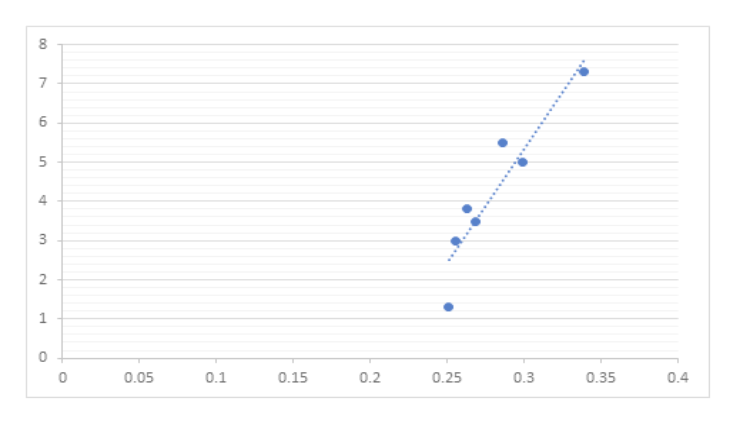In baseball, is there a linear correlation between batting average and home run percentage? Let x represent the batting average of a professional baseball player, and let y represent the player's home run percentage (number of home runs per 100 times at bat). A random sample of n = 7 professional baseball players gave the following information.x0.2510.2550.2860.2630.2680.3390.299y1.33.05.53.83.57.35.0(a) Make a scatter diagram of the data. Flash Player version 10 or higher is required for this question. You can get Flash Player free from Adobe's website. Then visualize the line you think best fits the data. (b) Use a calculator to verify that Σx = 1.961, Σx2 = 0.555, Σy = 29.4, Σy2 = 145.92 and Σxy = 8.571.Compute r. (Round to 3 decimal places.) As x increases, does the value of r imply that y should tend to increase or decrease? Explain your answer.Given our value of r, we can not draw any conclusions for the behavior of y as x increases.Given our value of r, y should tend to decrease as x increases.Given our value of r, y should tend to remain constant as x increases.Given our value of r, y should tend to increase as x increases.

Question

In baseball, is there a linear correlation between batting average and home run percentage? Let x represent the batting average of a professional baseball player, and let y represent the player's home run percentage (number of home runs per 100 times at bat). A random sample of n = 7 professional baseball players gave the following information.

 x 0.251 0.255 0.286 0.263 0.268 0.339 0.299 y 1.3 3 5.5 3.8 3.5 7.3 5
(a) Make a scatter diagram of the data.

Flash Player version 10 or higher is required for this question.
You can get Flash Player free from Adobe's website.

Then visualize the line you think best fits the data.

(b) Use a calculator to verify that Σx = 1.961, Σx2 = 0.555, Σy = 29.4, Σy2 = 145.92 and Σxy = 8.571.

Compute r. (Round to 3 decimal places.)

As x increases, does the value of r imply that y should tend to increase or decrease? Explain your answer.
Given our value of r, we can not draw any conclusions for the behavior of y as x increases.
Given our value of ry should tend to decrease as x increases.
Given our value of ry should tend to remain constant as x increases.
Given our value of ry should tend to increase as x increases.
Step 1

In this question we have the given data and using which we have to plot the scatter plot, and best line of fit and from given values we need to find the correlation coefficient and need to drew the conclusion about x and y.

Step 2

Part A) The scatter plot an...help_outlineImage Transcriptionclose8 6 5 2 0.05 0.2 0.1 0.15 0.25 0.3 0.35 0.4 st fullscreen

Want to see the full answer?

See Solution

Want to see this answer and more?

Our solutions are written by experts, many with advanced degrees, and available 24/7

See Solution
Tagged in

Other